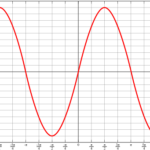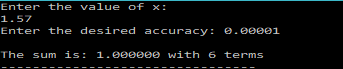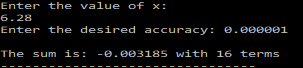# Evaluate Infinite Sine Series – C PROGRAM

In the last post I discussed, how to evaluate a Sine series for a given value of$x$ upto a certain number of terms.

In this post, I will show you how to modify that program to evaluate the sine series upto desired accuracy.To do that, instead of running the loop upto n,(to evaluate and sum the n terms) we would use a do-while loop which will run until the desired accuracy is reached.
That is till, the ratio:
accuracy=$\left|\frac{t_i}{sum} \right|$ becomes less or equal to the desired accuracy.

This will be our terminating condition for the do-while loop.

Therefore, the C program to find the sin(x), correct upto a given accuracy, can be written as shown below.

### PROGRAM:

/********************************
******INFINITE SERIES SUM**********
Series: sin(x) = x - (x^3/3!) + (x^5/5!) + .....
********************************/
#include<stdio.h>
#include<math.h>
main(){
int i=1;
double x,t0,t1,R,sum,eps;
printf("Enter the value of x:\n");
scanf("%lf",&x);
printf("Enter the desired accuracy: ");
scanf("%lf",&eps);
//Initialize First Term
t0=x;
//Make sum equal to the first term
sum=t0;
do{
//Find the ratio of the second term to the first term using already known relation
R=-(x*x)/(2*i+1)/(2*i);
//Calculate the second term
t1=R*t0;
//find the new sum
sum=sum+t1;
t0=t1;
i++;
//keep on summing terms until the required accuracy is reached
}while(fabs(t1/sum)>eps);
printf("\nThe sum is: %f with %d terms",sum,i);
}


### OUTPUT:[wpedon id="7041" align="center"]